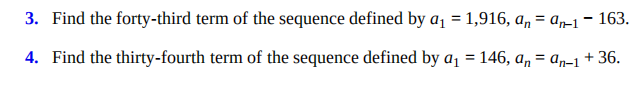### ¿Todavía tienes preguntas de matemáticas?

Pregunte a nuestros tutores expertos
Algebra
Pregunta3. Find the forty-third term of the sequence defined by $$a _ { 1 } = 1,916 , a _ { n } = a _ { n - 1 } - 163$$ . 4. Find the thirty-fourth term of the sequence defined by $$a _ { 1 } = 146 , a _ { n } = a _ { n - 1 } + 36$$ .

3. a2 = a1-163 = 1916-163 = 1753

d = 1753-1916 = -163, and a1=1916

the equation of a AP is an=a+d(n-1)

an=1916-163(n-1)

a43 = 1916-163(42) = -4930

4. a1=146, d=36

an=146+36(n-1)

a34 = 146+36(33) = 1334

Solución
View full explanation on CameraMath App.